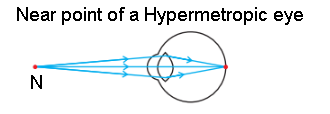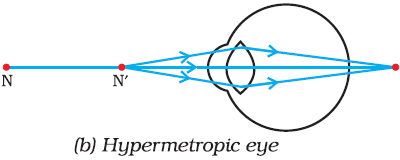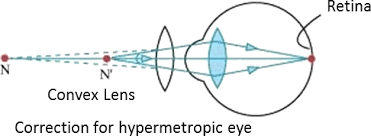3 Tutor System
Starting just at 265/hour

# Make a diagram to show how hypermetropia is corrected. The near point of a hypermetropia eye is 1 m. What is the power of the lens required to correct this defect? Assume that the near point of the normal eye is 25 cm.

It is corrected by using convex lens of suitable focal length.

Near point hypermetropia eye v = -1m = -100cm

Object distance u = -25cm
According to formula, $$\frac{1}{v} – \frac{1}{u} = \frac{1}{f}$$

Near point of hypermetropia eye:-
$$\frac{1}{f} = - \frac{1}{100} – \frac{1}{-25} = \frac{-1}{100} + \frac{1}{25}$$
$$\frac{1}{f} = \frac{(-1+4)}{(100)} = \frac{3}{100}$$
f = $$\frac{100}{3}$$

Near point of a hypermetropic eyeHypermetropia eye:-
f = $$\frac{100}{3}$$
$$P = \frac{1}{f} =\frac{ (3 x 100)}{(100)} = 3D$$

A convex lens of power +3.0 D is required to correct the defect.Correction of a hypermetropia eye